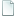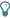##Topic: How to Make NWC Play Jazz (continued) (Read 10563 times)previous topic - next topic

How to Make NWC Play Jazz (continued)##### 2002-05-03 02:28 pm
Here is an addition to Marsu's tip "How to Make NWC Play Jazz" (https://forum.noteworthycomposer.com/?topic=2363). It is a simplified formula which gives a bunch of values for T1 and T2. This formula is T1 = n/(n+1) * T and T2 = n/(n-1) * T, with an arbitrary number for n.

Examples:
n=2: T1 = 2/3 * T, T2 = 2 * T; feeling = 3/4 + 1/4.
n=3: T1 = 3/4 * T, T2 = 3/2 * T; feeling = 4/6 + 2/6 = 2/3 + 1/3.
n=4: T1 = 4/5 * T, T2 = 4/3 * T; feeling = 5/8 + 3/8.
n=5: T1 = 5/6 * T, T2 = 5/4 * T; feeling = 6/10 + 4/10 = 3/5 + 2/5.
n=6: T1 = 6/7 * T, T2 = 6/5 * T; feeling = 7/12 + 5/12.
and so on.

Re: How to Make NWC Play Jazz (continued)